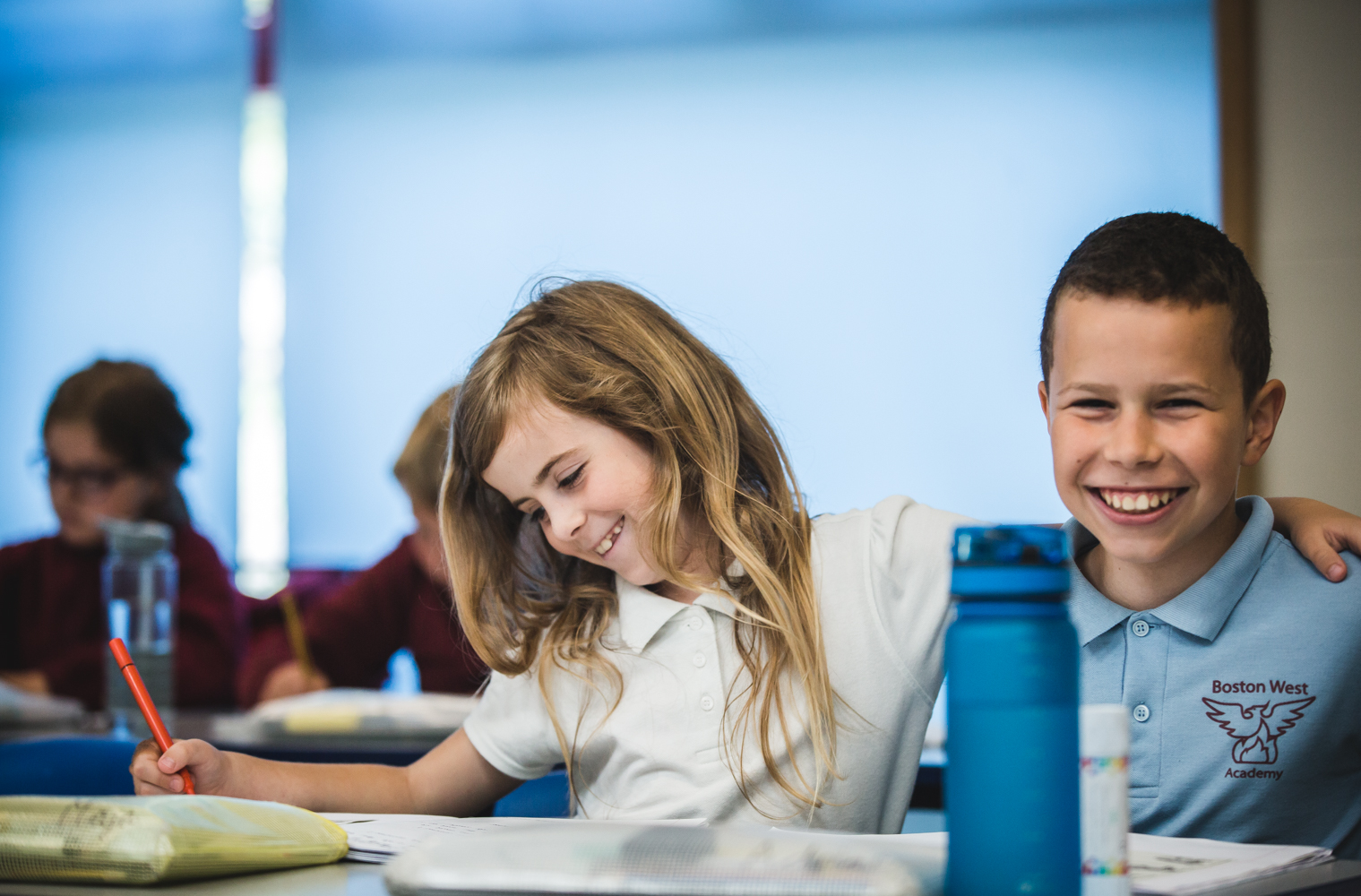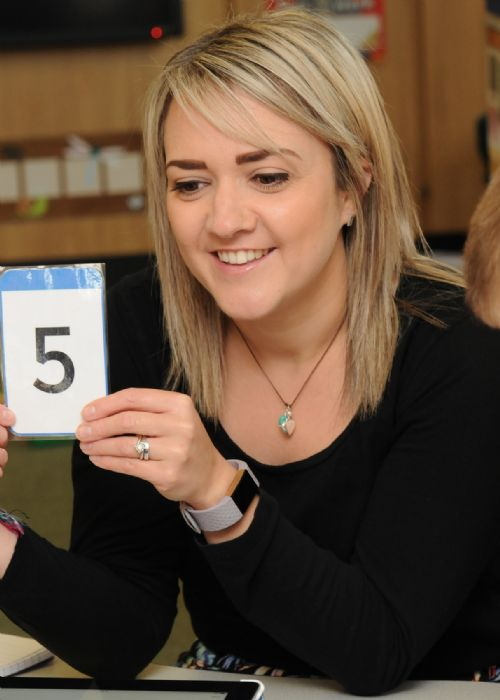•••••Proud to be part of

# Mathematics

###Mathematics is taught daily as a discrete subject, although opportunities are made, whenever possible, to apply knowledge and understanding across the curriculum.

In our school, mathematics is delivered using mastery approaches, which enable children to develop a secure understanding of key concepts in an engaging way.

To enable our children to grow as successful mathematicians, they are supported to develop: fluency in number facts, times tables and written methods of calculating; reasoning skills and problem solving strategies.

Children’s mathematical understanding is developed through the use of concrete apparatus and pictorial representations, before moving onto abstract concepts and calculations. Children are helped to acquire confidence, enthusiasm and resilience through oral and mental activities, talk tasks, investigations, practical tasks and mathematical games. In mathematics lessons children are frequently encouraged to explain their mathematical thinking using terminology correctly. When children demonstrate that they have a secure grasp of a new concept, they are given opportunities to apply this new learning in a range of different contexts.

### Mathematical vocabulary

The National Centre for Excellence in the Teaching of Mathematics has produced a useful glossary which gives definitions of the words and terms used in school when teaching mathematics.

### Our mathematics curriculumWe use White Rose Maths to support the planning and assessment of our mathematics curriculum. Below are the schemes of learning we use in each year group. These provide an overview of when key mathematical concepts are taught during the academic year.

We follow the White Rose Maths calculation policy for the 4 key operations of addition, subtraction, multiplication and division. However, this is currently under review. Below are the calculation policies from White Rose that we have been following as a school: